Archimedes' spiral

spiral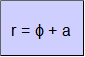The Archimedes' spiral (or spiral of Archimedes) is a kind of Archimedean spiral. Some authors define this spiral as the combination of the curves r = φ and r = -φ.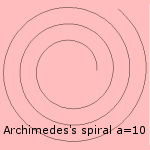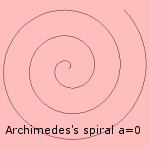Because there is a linear relation between radius and the angle, the distance between the windings is constant. There are two classical mathematical questions for which this spiral gives a simple solution: The quadrature of the circle was the quest to construct a square with the same area as a given circle. A first step would be to construct a line with a length π times the the length of a given line. When the Archimedes' spiral is available, this is easy: for an angle of magnitude π the radius is equal to this π. With the trisection was meant the quest to divide an angle in three equal parts. This is easy with the Archimedes' spiral, too. While the angle is linear with the radius, a division of the radius in n equal parts gives n equal angles in the spiral. The Greek - who found these construction methods - were not satisfied, however: they preferred to use no other construction tools than a ruler and a pair of compasses.The curve can be used to convert uniform angular motion into uniform linear motion: build a cam consisting of two branches of the spiral curve. Rotating this device with constant speed about its center results in a uniform linear motion at the crossing with a straight line through the center. This quality has been used in old sewing-machines (see picture).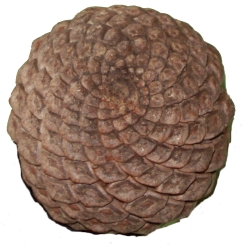In nature the curve can be seen for the pine-cone (see picture). Some ammonites have the form of a spiral of Archimedes, while most the form of a logarithmic spiral. The Archimedes' spiral describes a growth that just adds, in contrary to the logarithmic spiral, that grows related to its size. Three-dimensionally the curve is the orthogonal projection (on a plane perpendicular to the axis) of the spiral cone of Pappus. Some relations with other curves are the following: the curve is the pedal of the involute of a circle its inverse is the hyperbolic spiral the conchoid of the curve is also an Archimedes' spiral. It was of course Archimedes to explore this curve for the first time (225 BC). He wrote about it in his work 'on spirals'. It was his friend Conon who already considered the curve. Later it was Sacchi who studied the curve (in 1854).This spiral can be seen on the desktop at startup of your computer, when your friend has been affected by the win32.hybris virus. This email worm is able to extend its functionality by using plugins. One of these plugins is the one that displays an Archimedes' spiral, which is next to impossible to close. The branches for negative and positive φ can be combined, the resulting curve has been given several names: neoid, swan-like curve, streamline.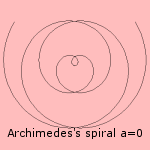The rectangular variant of this spiral is the rectangular Archimedes' spiral.